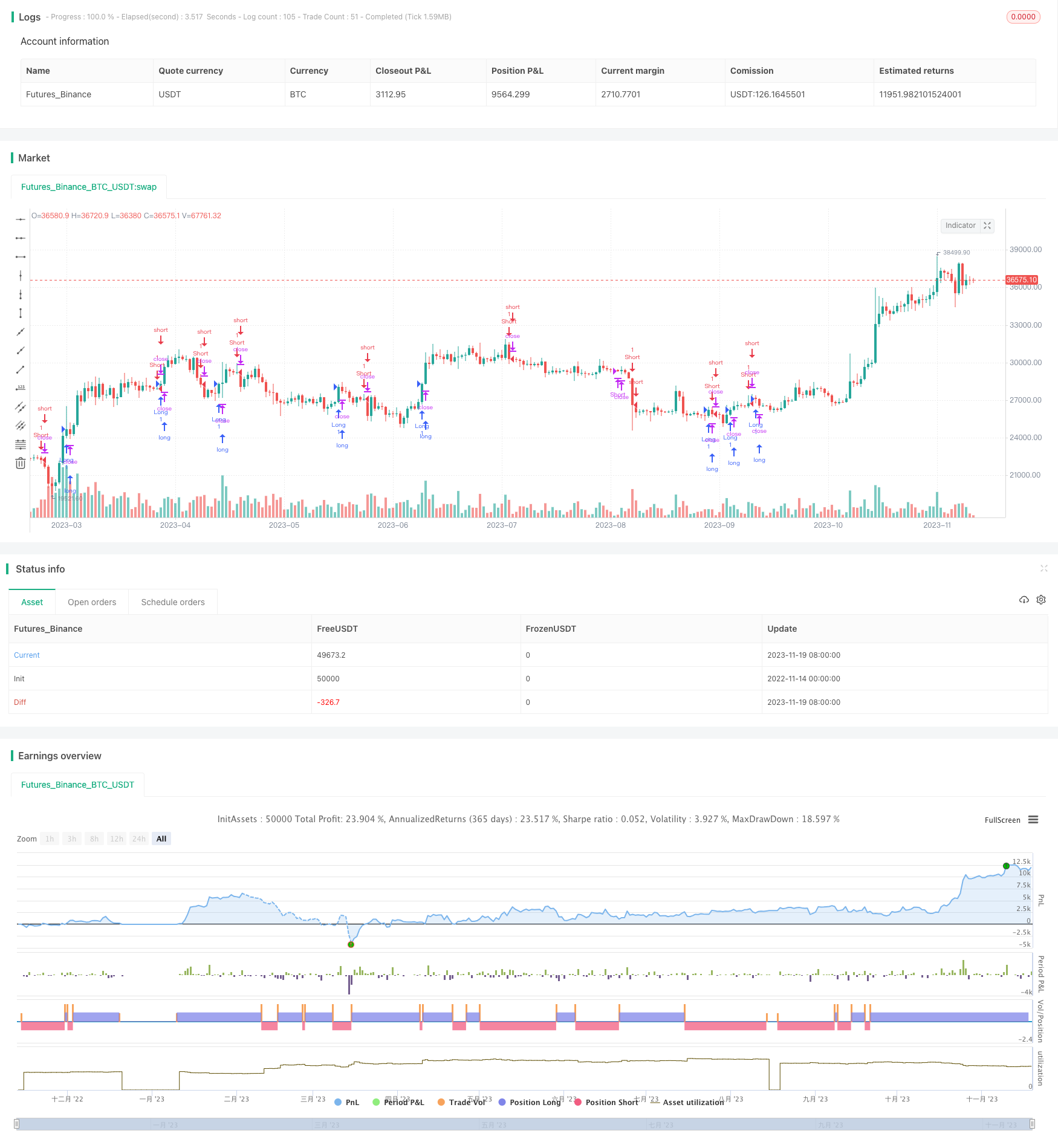Author: ChaoZhang, Date: 2023-11-21 11:11:51
Tags:## Overview

This strategy builds a trading volume model using moving average and standard deviation of trading volume, and determines the trend direction with the moving average of price to generate trading signals when volume is normal. It also sets upper and lower limits for trading volume to avoid wrong signals when volume is abnormal.

## Strategy Logic

The core logic is to build trading volume model and judge price trend.

• Calculate the 40-period moving average of volume vavg as the baseline
• Calculate the 40-period standard deviation of volume vsd as the normal fluctuation range
• Calculate the 5-period moving average of volume vavgn as the latest volume level
• Set lower limit of volume lowlimit as vavg minus 1 times vsd
• Set upper limit of volume uplimit as vavg plus 2 times vsd
2. Judge price trend
• Calculate the 20-period moving average of close price mavg as the indicator of price trend
• When mavg crosses above its previous day and vavgn is above lowlimit, go long
• When mavg crosses below its previous day and vavgn is above lowlimit, go short
• Close position when mavg trend reverses

The strategy combines trading volume model and price trend to avoid chasing price trends when volume is abnormal, which can filter out some false signals.

1. Combining volume changes to judge price trend can filter out some false signals and make the trading signals more reliable
2. Building trading volume model using standard deviation avoids extreme volume impact
3. Adjustable parameters of moving average can adapt to price changes in different cycles

## Risk Analysis

1. Volume and price may diverge in short term, leading to missing price trends
2. Improper parameter settings of volume may cause model failure
3. No stop loss in the strategy may lead to large losses

Solutions:

1. Adjust moving average parameters properly to optimize the model
2. Add stop loss logic to control single loss

## Optimization Directions

1. Add more indicators to judge price trend to make signals more reliable
2. Increase machine learning module to train parameters of volume and price models based on data
3. Add stop loss logic to prevent excessive single loss
4. Optimize entry logic to ensure higher probability of catching trends
5. Combine indicators like ATR to automatically adjust stop loss distance

## Summary

The overall logic of this strategy is clear, using volume to avoid chasing false trends and the entry signals are relatively reliable. But the strategy itself is simple with large room for expansion. By adding more indicators, machine learning, stop loss and other modules, it can further improve the stability and ability to catch trends. This is a typical trend chasing strategy. After optimization, it can become a very practical quantitative strategy.

```/*backtest
start: 2022-11-14 00:00:00
end: 2023-11-20 00:00:00
period: 1d
basePeriod: 1h
exchanges: [{"eid":"Futures_Binance","currency":"BTC_USDT"}]
*/

// This source code is subject to the terms of the Mozilla Public License 2.0 at https://mozilla.org/MPL/2.0/

//@version=4
strategy("交易量底部标准差系统", overlay=true)

options = input(1,'')
length = input(40,'')
nlow = input(5,'')
factor = input(1.0,'')

vavg = 0.0
vavgn = 0.0
vsd = 0.0
lowlimit = 0.0
uplimit = 0.0
mavg = 0.0
aror = 0.0
savevol = 0.0

//Find average volume, replacing bad values

if (volume != 0)
savevol := volume
else
savevol := savevol

// Replace high volume days because they distort standard deviation
if (adjvol > 2 * factor * nz(vsd))
else

// Extreme volume limits
lowlimit := vavg - factor * vsd
uplimit := vavg + 2 * factor * vsd

// System rules based on moving average trend
mavg := sma(close,length/2)

// Only enter on new trend signals
if (options == 2)
if (mavg > mavg and mavg <= mavg)
strategy.entry("Long", strategy.long)
if (mavg<mavg and mavg>=mavg)
strategy.entry("Short", strategy.short)
else
if (mavg > mavg and vavgn > lowlimit)
strategy.entry("Long", strategy.long)
if (mavg < mavg and vavgn > lowlimit)
strategy.entry("Short", strategy.short)

// Exit on low volume
if (options != 1)
if (mavg<mavg or (strategy.position_size > 0 and vavgn<= lowlimit))
strategy.close("Long")
if (mavg>mavg or (strategy.position_size > 0 and vavgn<= lowlimit))
strategy.close("Short")
else
if (mavg < mavg)
strategy.close("Long")
if (mavg > mavg)
strategy.close("Short")
```

More